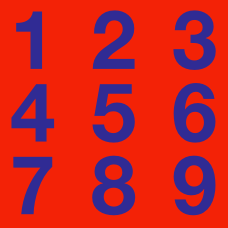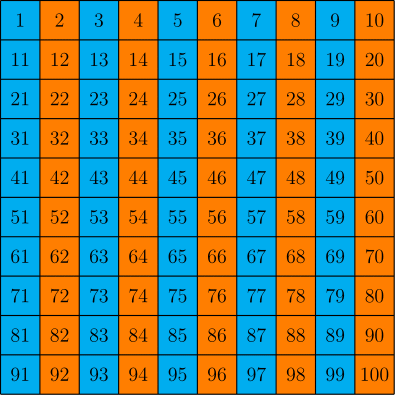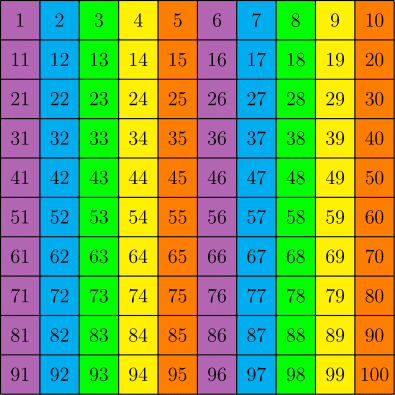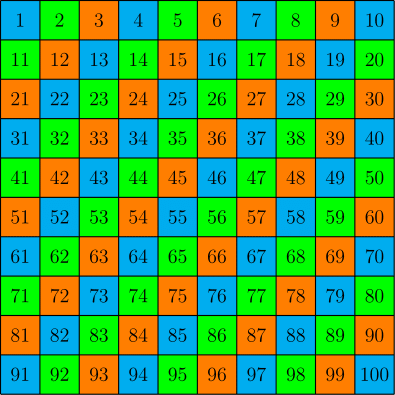Number Theory

# Modular Operation WarmupThe pattern above is blue-orange-blue-orange-blue-orange ... on to infinity.

If all integers are colored in the same pattern as the numbers in this grid, what color is 1234?The pattern above is blue-orange-blue-orange-blue-orange ... on to infinity. If you multiply a blue number by an orange number, what color will the product be?

Note: Assume that all integers are colored according to the same pattern as the numbers in the grid.The pattern is purple-blue-green-yellow-orange-purple-.... on to infinity. If you add a blue number to a yellow number, what color will the sum be?

Note: Assume that all integers are colored according to the same pattern as the numbers in the grid.The pattern is purple-blue-green-yellow-orange-purple-.... on to infinity. If you multiply a green number by a yellow number, what color will the product be?

Note: Assume that all integers are colored according to the same pattern as the numbers in the grid.The pattern is blue-green-orange-.... on to infinity. What color is $8 \times 17 \, ?$

Note: Assume that all integers are colored according to the same pattern as the numbers in the grid.

×